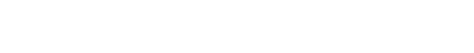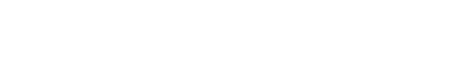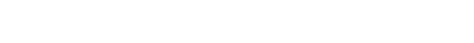# Power Factor Correction PFC Tutorial

In this Power Factor Correction PFC tutorial, a basic PFC circuit and the calculations used to design the circuit will be demonstrated.

A PFC circuit is required as the power factor in a system can be degraded.  One of these reasons is due to reactive power, the other is due to harmonics generated by the load device.  This tutorial will not cover harmonics, that will be covered in a later project build where an active PFC is designed and built around a Texas Instruments C2000 microprocessor.

Reactive power is a quantity normally only defined in alternating current (AC) systems, a countries national grid consists of an AC system.  Most AC systems consist of a sinusoidal wave with a frequency of 50 or 60Hz, the voltage and the current will both fluctuate at this frequency, but not necessarily at the same time.  The power being transmitted will fluctuate around an average value, assuming the voltage and current waveforms are in phase, then this value is known as the ‘real’ or ‘true’ power.  For a domestic household the real or true power over time, is measured by the electricity meter as kilowatt hours (kWh).

If the average value is zero then the voltage and current would be completely out of phase and the power being transmitted is said to be reactive power.  The unit for reactive power is the VAR, which stands for Volt Ampere Reactive.  Depending by how much the voltage and current sinusoids are out of phase, determines the amount of reactive power verses real power, and the resultant power factor.  There is also one other element to consider, this is known as the ‘apparent’ power.  Apparent power is the power that appears to be flowing to the load.  The equations below will help clarify the relationship between these terms, real or true power is denoted by P, reactive power by Q and apparent by S.Reactive power in a system is caused when the load device in a system stores power, this power then flows back towards the primary power source.

The inductive and capacitive loads affect on the current wave form is known as the Displacement Power Factor.  The harmonic distortion caused by switching elements, is known as the Total Harmonic Distortion or THD.  Both the Displacement Power Factor and the THD are used to calculate the overall power factor.  In the following example a simplified model is over viewed where the THD is assumed to be zero.

## Basic PFC Circuit

The video below demonstrates a basic AC circuit, under 3 conditions;

1. The circuits first condition is with a purely resistive load, and the voltage and current waveforms can be observed to be in phase.
2. The next condition an inductive load is switched in parallel with the resistive load, the current sine wave can be observed to become out of phase with the voltage.
3. The third and final condition is to place a capacitor in parallel with the inductor and resistor, this brings the current sine wave back in phase with the voltage.For the basic resistor circuit it is fairly straight forward to calculate the power in the circuit.The image below shows the circuit again, the yellow trace is the current and blue trace is the voltage.  The peak to peak and rms values for the traces can be seen to the right of the oscilloscope screen, bearing in mind that the current transformer is set to 1mV/mA, the values can be seen to almost mirror the calculations above.To calculate the power when the inductor has been added, requires the inductor reactance to be calculated, which then allows the total impedance of the circuit to be determined. Complex numbers will be used to determine the inductor reactance and the total circuit impedance.Using the basic parallel calculation in rectangular form, the impedance can be calculated and then the new circuit current.As with the previous calculation for the resistive load, the inductor calculation can be compared with the image below showing the oscilloscope trace and values.From this the circuits displacement power factor can also be determined, using the resultant phase shift of the current due to the new inductive load.With the power factor calculated, it is now possible to determine the real power, apparent power and reactive power.The final part of this tutorial involves calculating the value of the power factor correction capacitor.  It can be seen from the video this brings the current waveform back in phase with the voltage waveform.  The capacitor achieves this by cancelling the inductors reactance out, this makes the load appear purely resistive.  The calculation for this is as follows.Furthermore by using the resonance formulae, it can be seen that the inductor and capacitor are in resonance.The power factor is now effectively 1 as there is no phase difference between the voltage and current waveforms.  It can be shown that the same real power is still being delivered to the load.As with the previous calculations the image below can be seen to match the equation.Further reading on power factor can also be found here.

## 5 thoughts on “Power Factor Correction PFC Tutorial”

Hi Ant
Great posts, I just discovered, and I will read more
For your problems about fixed point and FPU, try the F28069M or the new F28377S
they are 25 and 30 USD, so not expensive at all and still compatible with Launchpad XL, so you can unplug and plug on your Power Electronics boards.
Hope it helps
Best regards
Lotfi

Hi Lotfi,

Thanks, yep a faster MCU would definitely be one way to solve the the issue, improving my code is definitely the first step though. Thanks for the link I didn’t know TI had brought out some new Launchpad variations.

Cheers,
Ant

HI can you make a tutorial a bout active PFC with c2000 launchpad? This tutorial is very great for me. thanks

Hi,

Something I am working on but won’t be available for awhile as I need to rebuild the prototype and rewrite the algorithm using fixed point. I originally used the C2000 Launchpad, my code was based in C, using floating point numbers, however the algorithm could not execute fast enough and my PWM frequency was reduced to 30kHz (originally planned for 60kHz). The system was effectively tracking the zero crossing point, the duty cycle was increasing from the zero crossing to the maximum current and then decreasing from the maximum current back to the zero crossing point, as it should. But the PWM frequency was simply not fast enough due to the speed of my algorithm’s execution time, inside the ADC interrupt, this was due to me using floating point and the C language when fixed point and Assembly is used in most examples I have seen. I need to move the code over to fixed point and probably use a 120MHz C2000 CPU as I have little knowledge of Assembly language.

Have you looked into a C2000 controlled PFC and how far along are you?

Best regards,

Ant